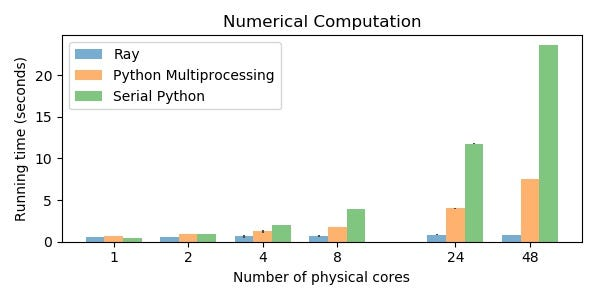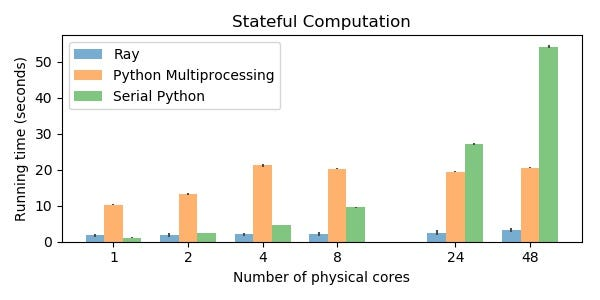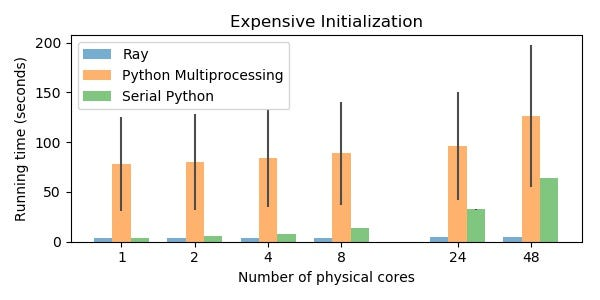# Faster Python without restructuring your code10x Faster Parallel Python Without Python Multiprocessing

While Python’s multiprocessing library has been used successfully for a wide range of applications, in this blog post, we show that it falls short for several important classes of applications including numerical data processing, stateful computation, and computation with expensive initialization. There are two main reasons:

• Inefficient handling of numerical data.
• Missing abstractions for stateful computation (i.e., an inability to share variables between separate “tasks”).

Ray is a fast, simple framework for building and running distributed applications that addresses these issues. For an introduction to some of the basic concepts, see this blog post. Ray leverages Apache Arrow for efficient data handling and provides task and actor abstractions for distributed computing.

This blog post benchmarks three workloads that aren’t easily expressed with Python multiprocessing and compares Ray, Python multiprocessing, and serial Python code. Note that it’s important to always compare to optimized single-threaded code.

In these benchmarks, Ray is 10–30x faster than serial Python, 5–25x faster than multiprocessing, and 5–15x faster than the faster of these two on a large machine.The benchmarks were run on EC2 using the m5 instance types (m5.large for 1 physical core and m5.24xlarge for 48 physical cores). Code for running all of the benchmarks is available here. Abbreviated snippets are included in this post. The main differences are that the full benchmarks include 1) timing and printing code, 2) code for warming up the Ray object store, and 3) code for adapting the benchmark to smaller machines.

### Benchmark 1: Numerical Data

Many machine learning, scientific computing, and data analysis workloads make heavy use of large arrays of data. For example, an array may represent a large image or dataset, and an application may wish to have multiple tasks analyze the image. Handling numerical data efficiently is critical.

Each pass through the for loop below takes 0.84s with Ray, 7.5s with Python multiprocessing, and 24s with serial Python (on 48 physical cores). This performance gap explains why it is possible to build libraries like Modin on top of Ray but not on top of other libraries.

The code looks as follows with Ray.

``````import numpy as np
import psutil
import ray
import scipy.signal

num_cpus = psutil.cpu_count(logical=False)

ray.init(num_cpus=num_cpus)

@ray.remote
def f(image, random_filter):
# Do some image processing.
return scipy.signal.convolve2d(image, random_filter)[::5, ::5]

filters = [np.random.normal(size=(4, 4)) for _ in range(num_cpus)]

# Time the code below.

for _ in range(10):
image = np.zeros((3000, 3000))
image_id = ray.put(image)
ray.get([f.remote(image_id, filters[i]) for i in range(num_cpus)])``````

parallel_python_ray_numerical_computation.py

By calling `ray.put(image)`, the large array is stored in shared memory and can be accessed by all of the worker processes without creating copies. This works not just with arrays but also with objects that contain arrays (like lists of arrays).

When the workers execute the `f` task, the results are again stored in shared memory. Then when the script calls `ray.get([...])`, it creates numpy arrays backed by shared memory without having to deserialize or copy the values.

These optimizations are made possible by Ray’s use of Apache Arrow as the underlying data layout and serialization format as well as the Plasma shared-memory object store.

The code looks as follows with Python multiprocessing.

``````from multiprocessing import Pool
import numpy as np
import psutil
import scipy.signal

num_cpus = psutil.cpu_count(logical=False)

def f(args):
image, random_filter = args
# Do some image processing.
return scipy.signal.convolve2d(image, random_filter)[::5, ::5]

pool = Pool(num_cpus)

filters = [np.random.normal(size=(4, 4)) for _ in range(num_cpus)]

# Time the code below.

for _ in range(10):
image = np.zeros((3000, 3000))
pool.map(f, zip(num_cpus * [image], filters))``````

parallel_python_multiprocessing_numerical_computation.py

The difference here is that Python multiprocessing uses pickle to serialize large objects when passing them between processes. This approach requires each process to create its own copy of the data, which adds substantial memory usage as well as overhead for expensive deserialization, which Ray avoids by using the Apache Arrow data layout for zero-copy serialization along with the Plasma store.

### Benchmark 2: Stateful Computation

Workloads that require substantial “state” to be shared between many small units of work are another category of workloads that pose a challenge for Python multiprocessing. This pattern is extremely common, and I illustrate it hear with a toy stream processing application.State is often encapsulated in Python classes, and Ray provides an actor abstraction so that classes can be used in the parallel and distributed setting. In contrast, Python multiprocessing doesn’t provide a natural way to parallelize Python classes, and so the user often needs to pass the relevant state around between `map` calls. This strategy can be tricky to implement in practice (many Python variables are not easily serializable) and it can be slow when it does work.

Below is a toy example that uses parallel tasks to process one document at a time, extract the prefixes of each word, and return the most common prefixes at the end. The prefix counts are stored in the actor state and mutated by the different tasks.

This example takes 3.2s with Ray, 21s with Python multiprocessing, and 54s with serial Python (on 48 physical cores).

The Ray version looks as follows.

``````from collections import defaultdict
import numpy as np
import psutil
import ray

num_cpus = psutil.cpu_count(logical=False)

ray.init(num_cpus=num_cpus)

@ray.remote
class StreamingPrefixCount(object):
def __init__(self):
self.prefix_count = defaultdict(int)
self.popular_prefixes = set()

for word in document:
for i in range(1, len(word)):
prefix = word[:i]
self.prefix_count[prefix] += 1
if self.prefix_count[prefix] > 3:

def get_popular(self):
return self.popular_prefixes

streaming_actors = [StreamingPrefixCount.remote() for _ in range(num_cpus)]

# Time the code below.

for i in range(num_cpus * 10):
document = [np.random.bytes(20) for _ in range(10000)]

# Aggregate all of the results.
results = ray.get([actor.get_popular.remote() for actor in streaming_actors])
popular_prefixes = set()
for prefixes in results:
popular_prefixes |= prefixes``````

parallel_python_ray_stateful_computation.py

Ray performs well here because Ray’s abstractions fit the problem at hand. This application needs a way to encapsulate and mutate state in the distributed setting, and actors fit the bill.

The multiprocessing version looks as follows.

``````from collections import defaultdict
from multiprocessing import Pool
import numpy as np
import psutil

num_cpus = psutil.cpu_count(logical=False)

def accumulate_prefixes(args):
running_prefix_count, running_popular_prefixes, document = args
for word in document:
for i in range(1, len(word)):
prefix = word[:i]
running_prefix_count[prefix] += 1
if running_prefix_count[prefix] > 3:
return running_prefix_count, running_popular_prefixes

pool = Pool(num_cpus)

running_prefix_counts = [defaultdict(int) for _ in range(4)]
running_popular_prefixes = [set() for _ in range(4)]

for i in range(10):
documents = [[np.random.bytes(20) for _ in range(10000)]
for _ in range(num_cpus)]
results = pool.map(
accumulate_prefixes,
zip(running_prefix_counts, running_popular_prefixes, documents))
running_prefix_counts = [result for result in results]
running_popular_prefixes = [result for result in results]

popular_prefixes = set()
for prefixes in running_popular_prefixes:
popular_prefixes |= prefixes``````

parallel_python_multiprocessing_stateful_computation.py

The challenge here is that `pool.map` executes stateless functions meaning that any variables produced in one `pool.map` call that you want to use in another `pool.map` call need to be returned from the first call and passed into the second call. For small objects, this approach is acceptable, but when large intermediate results needs to be shared, the cost of passing them around is prohibitive (note that this wouldn’t be true if the variables were being shared between threads, but because they are being shared across process boundaries, the variables must be serialized into a string of bytes using a library like pickle).

Because it has to pass so much state around, the multiprocessing version looks extremely awkward, and in the end only achieves a small speedup over serial Python. In reality, you wouldn’t write code like this because you simply wouldn’t use Python multiprocessing for stream processing. Instead, you’d probably use a dedicated stream-processing framework. This example shows that Ray is well-suited for building such a framework or application.

One caveat is that there are many ways to use Python multiprocessing. In this example, we compare to `Pool.map` because it gives the closest API comparison. It should be possible to achieve better performance in this example by starting distinct processes and setting up multiple multiprocessing queues between them, however that leads to a complex and brittle design.

### Benchmark 3: Expensive Initialization

In contrast to the previous example, many parallel computations don’t necessarily require intermediate computation to be shared between tasks, but benefit from it anyway. Even stateless computation can benefit from sharing state when the state is expensive to initialize.

Below is an example in which we want to load a saved neural net from disk and use it to classify a bunch of images in parallel.This example takes 5s with Ray, 126s with Python multiprocessing, and 64s with serial Python (on 48 physical cores). In this case, the serial Python version uses many cores (via TensorFlow) to parallelize the computation and so it is not actually single threaded.

Suppose we’ve initially created the model by running the following.

``````import tensorflow as tf

x_train, y_train = mnist
x_train = x_train / 255.0
model = tf.keras.models.Sequential([
tf.keras.layers.Flatten(input_shape=(28, 28)),
tf.keras.layers.Dense(512, activation=tf.nn.relu),
tf.keras.layers.Dropout(0.2),
tf.keras.layers.Dense(10, activation=tf.nn.softmax)
])
model.compile(
loss='sparse_categorical_crossentropy',
metrics=['accuracy'])
# Train the model.
model.fit(x_train, y_train, epochs=1)
# Save the model to disk.
filename = '/tmp/model'
model.save(filename)``````

save_model.py

Now we wish to load the model and use it to classify a bunch of images. We do this in batches because in the application the images may not all become available simultaneously and the image classification may need to be done in parallel with the data loading.

The Ray version looks as follows.

``````import psutil
import ray
import sys
import tensorflow as tf

num_cpus = psutil.cpu_count(logical=False)

ray.init(num_cpus=num_cpus)

filename = '/tmp/model'

@ray.remote
class Model(object):
def __init__(self, i):
# Pin the actor to a specific core if we are on Linux to prevent
# contention between the different actors since TensorFlow uses
if sys.platform == 'linux':
psutil.Process().cpu_affinity([i])
# Load the model and some data.
self.x_test = mnist / 255.0

def evaluate_next_batch(self):
# Note that we reuse the same data over and over, but in a
# real application, the data would be different each time.
return self.model.predict(self.x_test)

actors = [Model.remote(i) for i in range(num_cpus)]

# Time the code below.

# Parallelize the evaluation of some test data.
for j in range(10):
results = ray.get([actor.evaluate_next_batch.remote() for actor in actors])``````

parallel_python_ray_expensive_initialization.py

Loading the model is slow enough that we only want to do it once. The Ray version amortizes this cost by loading the model once in the actor’s constructor. If the model needs to be placed on a GPU, then initialization will be even more expensive.

The multiprocessing version is slower because it needs to reload the model in every map call because the mapped functions are assumed to be stateless.

The multiprocessing version looks as follows. Note that in some cases, it is possible to achieve this using the `initializer` argument to `multiprocessing.Pool`. However, this is limited to the setting in which the initialization is the same for each process and doesn’t allow for different processes to perform different setup functions (e.g., loading different neural network models), and doesn’t allow for different tasks to be targeted to different workers.

``````from multiprocessing import Pool
import psutil
import sys
import tensorflow as tf

num_cpus = psutil.cpu_count(logical=False)

filename = '/tmp/model'

def evaluate_next_batch(i):
# Pin the process to a specific core if we are on Linux to prevent
# contention between the different processes since TensorFlow uses
if sys.platform == 'linux':
psutil.Process().cpu_affinity([i])
x_test = mnist / 255.0
return model.predict(x_test)

pool = Pool(num_cpus)

for _ in range(10):
pool.map(evaluate_next_batch, range(num_cpus))``````

parallel_python_multiprocessing_expensive_initialization.py

What we’ve seen in all of these examples is that Ray’s performance comes not just from its performance optimizations but also from having abstractions that are appropriate for the tasks at hand. Stateful computation is important for many many applications, and coercing stateful computation into stateless abstractions comes at a cost.

### Run the Benchmarks

Before running these benchmarks, you will need to install the following.

``pip install numpy psutil ray scipy tensorflow``

If you have trouble installing `psutil`, then try using Anaconda Python.

The original benchmarks were run on EC2 using the m5 instance types (m5.large for 1 physical core and m5.24xlarge for 48 physical cores).

In order to launch an instance on AWS or GCP with the right configuration, you can use the Ray autoscaler and run the following command.

``````pip install numpy psutil ray scipy tensorflow
``````

An example `config.yaml` is provided here (for starting an m5.4xlarge instance).

While this blog post focuses on benchmarks between Ray and Python multiprocessing, an apples-to-apples comparison is challenging because these libraries are not very similar. Differences include the following.

• Ray is designed for scalability and can run the same code on a laptop as well as a cluster (multiprocessing only runs on a single machine).
• Ray workloads automatically recover from machine and process failures.
• Ray is designed in a language-agnostic manner and has preliminary support for Java.

## Basic Data Types in Python | Python Web Development For Beginners

In the programming world, Data types play an important role. Each Variable is stored in different data types and responsible for various functions. Python had two different objects, and They are mutable and immutable objects.

## How To Compare Tesla and Ford Company By Using Magic Methods in Python

Magic Methods are the special methods which gives us the ability to access built in syntactical features such as ‘<’, ‘>’, ‘==’, ‘+’ etc.. You must have worked with such methods without knowing them to be as magic methods. Magic methods can be identified with their names which start with __ and ends with __ like __init__, __call__, __str__ etc. These methods are also called Dunder Methods, because of their name starting and ending with Double Underscore (Dunder).

## Python Programming: A Beginner’s Guide

Python is an interpreted, high-level, powerful general-purpose programming language. You may ask, Python’s a snake right? and Why is this programming language named after it?

## Hire Python Developers

Are you looking for experienced, reliable, and qualified Python developers? If yes, you have reached the right place. At **[HourlyDeveloper.io](https://hourlydeveloper.io/ "HourlyDeveloper.io")**, our full-stack Python development services...

## Python any: How to Check If Element is Iterable or Not

Python any() function returns True if any element of an iterable is True otherwise any() function returns False. The syntax is any().